# Test: Electronic Devices - 3

## 10 Questions MCQ Test RRB JE for Electronics & Communication Engineering | Test: Electronic Devices - 3

Description
Attempt Test: Electronic Devices - 3 | 10 questions in 30 minutes | Mock test for Electronics and Communication Engineering (ECE) preparation | Free important questions MCQ to study RRB JE for Electronics & Communication Engineering for Electronics and Communication Engineering (ECE) Exam | Download free PDF with solutions
QUESTION: 1

### The open-circuit p-n junction diode without any Biasing is shown if NA = 2 × 1017/cm3, ND = 5 × 1016/cm3 then the depletion region width (W) is ______μm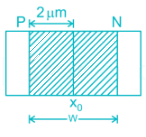Solution:

For the p-n junction diode, the depletion region width is given by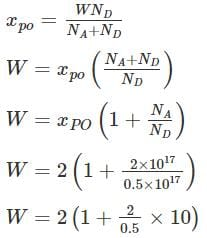W = 10 μm

*Answer can only contain numeric values
QUESTION: 2

### For the diode circuit shown below, If Vs = 24 sin 100πt, and the diode is practical with a cut in voltage of 0.7 V. The conduction angle of the circuit is _______ Degrees.

Solution:

For practical diode

When

Vi > 12 + 0.7 = 12.7 diode conducts

i.e

24 sin θ >12.7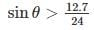θ > 31.93

Conduction angle = 180 – 2 (31.93)

= 116.12°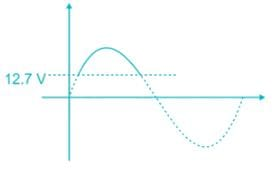QUESTION: 3

###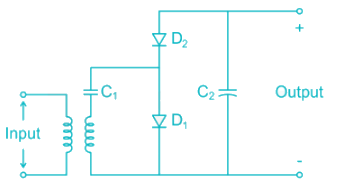The correct output waveform for the circuit shown if the input is a sinusoidal signal of maximum Amplitude Vmax is

Solution:

The circuit shown in a half-wave voltage double During Positive half cycle C1 and D1 Conducts and C is charged to Vmax During Negative half cycle C2 and D2conducts and

Vout = Vmax + VCl

= 2 Vmax

*Answer can only contain numeric values
QUESTION: 4

In the circuit shown, the forward biased LED has a voltage drop of 1.5 volts. If the battery voltage is 6V. Then the power displaced in the resistor R in milliwatts is _________mW. Current through LED is 15mA.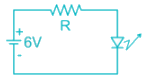Solution:

If r is the internal resistance of LED

Total current =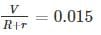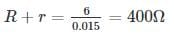As the drop across LED = 1.5 V

Internal resistance r =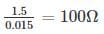The external resistance to be connected

R = 400 – 100 = 300Ω

Power dissipated in the resistor

I2R = 67.5 mW

QUESTION: 5

The voltage regulator circuit using a Zener diode is shown. The Zener diode current is limited in the range 5 ≤ iz ≤ 100 mA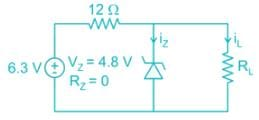The range of load resistance is

Solution: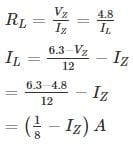IZ = (125 - IZ)mA

Case A:

IZ = 5 mA

IZ = 120 mA

= 0.12 A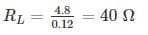Case B:

IZ = 100 mA

IZ = (125 – 100) mA

= 25 mA

= 0.025 A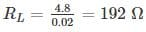*Answer can only contain numeric values
QUESTION: 6

For a P±n Si junction the reverse current at room temperature is 0.9 nA/cm2. If donor density is 1015 cm-3 and intrinsic carrier concentration is 1.05 × 1010. The minority carrier life time is ________ n sec.

[Assume μP = 450 cm2/V-sec, kT = 25mV ]

Solution:

For P±n junction the current density is given by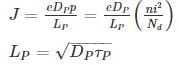Substituting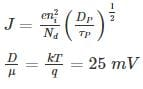DP = 0.025 × 450

= 11.25

τp = 4.32 × 10-9 sec

QUESTION: 7

The sketch of output voltage Vo vs. input voltage VI is of from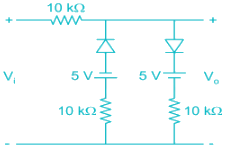Solution:

For voltages -5V < VI < 5 V both the diodes are off since the diodes are reverse biased.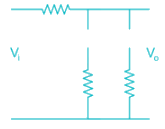The output voltage Vo = VI

For VI ≤ -5V

The diode D1 is forward biased, while D2 is reverse biased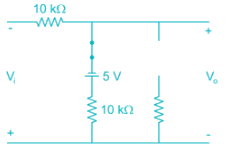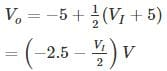For VI ≥ 5 V

The diode D1 is reverse biased, while D2 is forward biased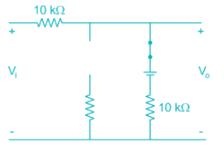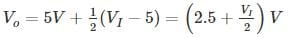Drawing sketch of Vo Vs VI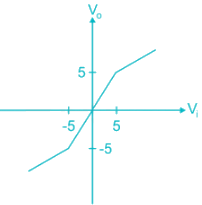*Answer can only contain numeric values
QUESTION: 8

Consider a pn junction at zero bias with an electric distribution as sketched below. Calculate the built-in-potential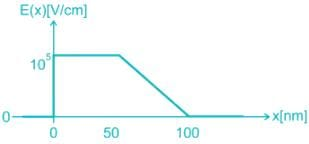Solution:

The built in potential is the integral of election field at zero bais: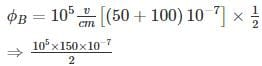⇒ 75 × 10-2 V

⇒ 0.75 V

QUESTION: 9

The correct waveform of the output of the given circuit is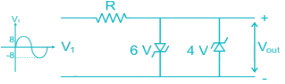Assume the Zener diodes are ideal with threshold voltage Vk = 0.7

Solution:

For positive cycle when VI = 0.7 V, the 6 V Zener diode is forward biased while 4 V is reverse biased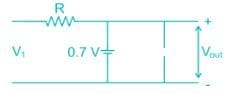For negative cycle VI <-0.7 V, the 6 V Zener diode is reverse biased 4 V Zener diode is forward biased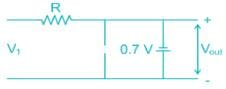For -0.7 < VI < 0.7 both the diodes are reverse biased hence Vout = VI

The output waveform will be of form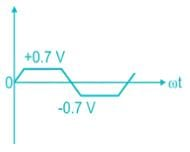QUESTION: 10

The correct statement regarding depletion and diffusion capacitance is

i) Depletion capacitance is dominant in reverse-bias voltage

ii) Diffusion capacitance is dominant in reverse-bias voltage

iii) The diffusion capacitance is due to a stored charge of minority electrons and minority holes near the depletion region

iv) Depletion capacitance is directly proportional to the width of the depletion region

Solution:

Diffusion capacitance is due to transfer of minority carries during forward bias. The minority carries diffuse from one end of junction to other, causing variation of charge with applied voltage. This leads to capacitance, it is present only in forward bias and is significantly higher than depletion capacitance in forward bias.

Depletion capacitance is due to storage of charges in reverse bias junction, which acts like parallel plate capacitance with forward voltage, the depletion width decreases increasing depletion capacitance. It is less than diffusion capacitance in forward bias mode.Use Code STAYHOME200 and get INR 200 additional OFF Use Coupon Code